/

### Function Description

Returns a two element array containing as its first termand its second term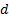which is the canonically simplified form of the input fraction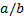where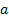and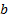are both integral and the input is in the form of a two element array consisting ofandrespectively.

The canonically simplified form of such a fraction is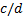whereis positive and the greatest common divisor of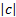andis 1.

Contents | Prev | Next